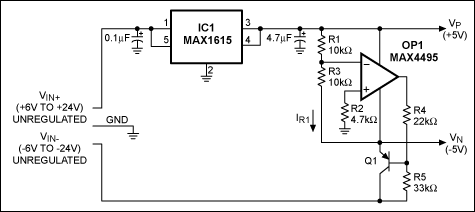# 利用空闲运放产生稳定的负电源

### AbstractCH1 = 稳定的-5V输出
CH2 = 负电源电压
CH3 = 稳定的+5V输出
CH4 = 正电源电压

VOP1+ = VOP1-
VOP1+ = 0V

VOP1-' = VP(R3/(R3+R1)
VOP1-'' = (VN(R1/(R1+R3)
VOP1- = VOP1-' + VOP1-''
VOP1- = VP(R3/(R3+R1)) + VN(R1/(R1+R3))
VN = -VP(R3/(R3+R1))((R1+R3)/R1)

VN = -VP(R3/R1)

IR1 = VP/R1

VN = -IR1 × R3 = (-VP/R1)R3